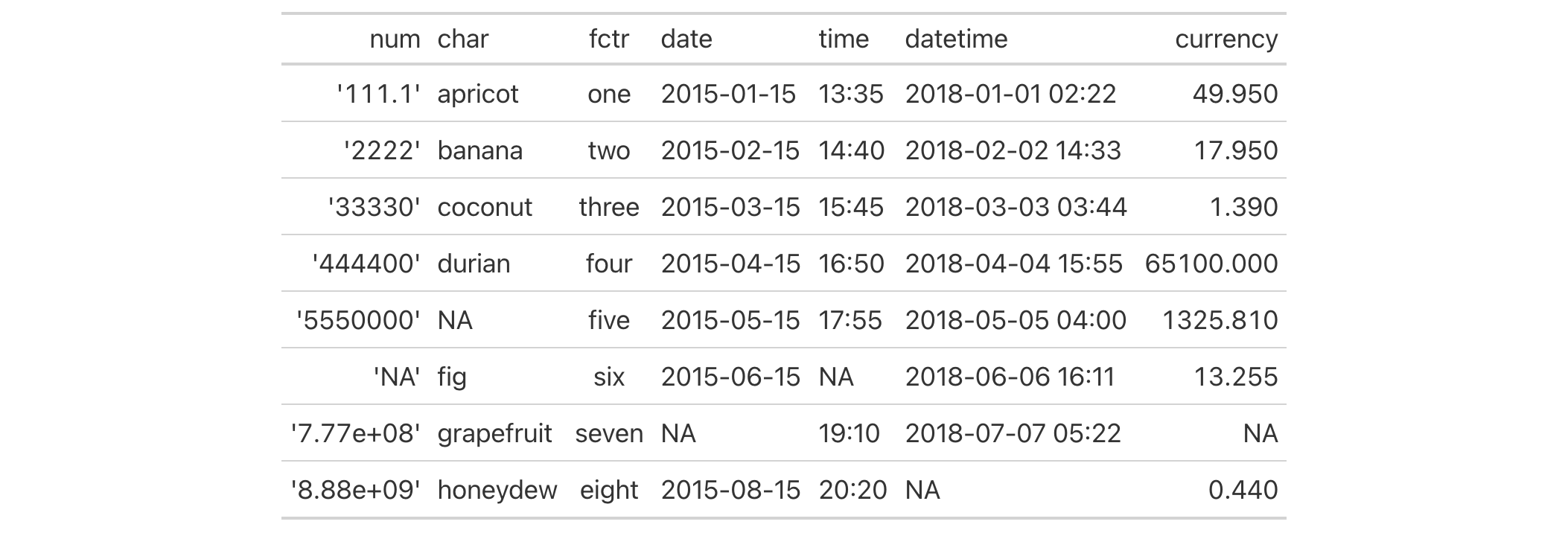# fmt: Set a column format with a formatter function In gt: Easily Create Presentation-Ready Display Tables

 fmt R Documentation

## Set a column format with a formatter function

### Description

The `fmt()` function provides a way to execute custom formatting functionality with raw data values in a way that can consider all output contexts.

Along with the `columns` and `rows` arguments that provide some precision in targeting data cells, the `fns` argument allows you to define one or more functions for manipulating the raw data.

If providing a single function to `fns`, the recommended format is in the form: `fns = function(x) ...`. This single function will format the targeted data cells the same way regardless of the output format (e.g., HTML, LaTeX, RTF).

If you require formatting of `x` that depends on the output format, a list of functions can be provided for the `html`, `latex`, `rtf`, and `default` contexts. This can be in the form of `fns = list(html = function(x) ..., latex = function(x) ..., default = function(x) ...)`. In this multiple-function case, we recommended including the `default` function as a fallback if all contexts aren't provided.

### Usage

```fmt(data, columns = everything(), rows = everything(), fns)
```

### Arguments

 `data` A table object that is created using the `gt()` function. `columns` The columns to format. Can either be a series of column names provided in `c()`, a vector of column indices, or a helper function focused on selections. The select helper functions are: `starts_with()`, `ends_with()`, `contains()`, `matches()`, `one_of()`, `num_range()`, and `everything()`. `rows` Optional rows to format. Providing `everything()` (the default) results in all rows in `columns` being formatted. Alternatively, we can supply a vector of row captions within `c()`, a vector of row indices, or a helper function focused on selections. The select helper functions are: `starts_with()`, `ends_with()`, `contains()`, `matches()`, `one_of()`, `num_range()`, and `everything()`. We can also use expressions to filter down to the rows we need (e.g., `[colname_1] > 100 & [colname_2] < 50`). `fns` Either a single formatting function or a named list of functions.

### Value

An object of class `gt_tbl`.

### Targeting the values to be formatted

Targeting of values is done through `columns` and additionally by `rows` (if nothing is provided for `rows` then entire columns are selected). Conditional formatting is possible by providing a conditional expression to the `rows` argument. See the Arguments section for more information on this.

### Examples

Use `exibble` to create a gt table. Format the numeric values in the `num` column with a function supplied to the `fns` argument.

```exibble %>%
dplyr::select(-row, -group) %>%
gt() %>%
fmt(
columns = num,
fns = function(x) {
paste0("'", x * 1000, "'")
}
)
```### Function ID

3-17

Other data formatting functions: `data_color()`, `fmt_bytes()`, `fmt_currency()`, `fmt_datetime()`, `fmt_date()`, `fmt_duration()`, `fmt_engineering()`, `fmt_fraction()`, `fmt_integer()`, `fmt_markdown()`, `fmt_number()`, `fmt_partsper()`, `fmt_passthrough()`, `fmt_percent()`, `fmt_roman()`, `fmt_scientific()`, `fmt_time()`, `sub_large_vals()`, `sub_missing()`, `sub_small_vals()`, `sub_values()`, `sub_zero()`, `text_transform()`